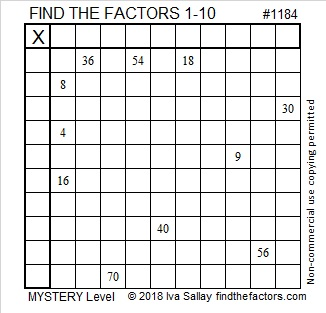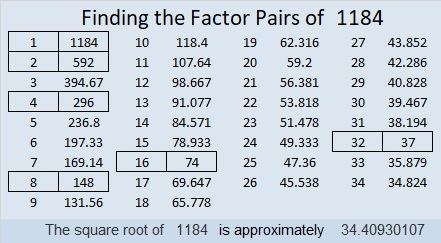# 1184 Mystery Level

The sets of clues in this Mystery Level puzzle have more than one possible common factor. Don’t guess which one you should use. In each case, the right factor can be selected by using logic. Will you figure out where all the factors from 1 to 10 go?Print the puzzles or type the solution in this excel file: 10-factors-1174-1186

Here are a few facts about the number 1184:

• 1184 is a composite number.
• Prime factorization: 1184 = 2 × 2 × 2 × 2 × 2 × 37, which can be written 1184 = 2⁵ × 37
• The exponents in the prime factorization are 5 and 1. Adding one to each and multiplying we get (5 + 1)(1 + 1) = 6 × 2 = 12. Therefore 1184 has exactly 12 factors.
• Factors of 1184: 1, 2, 4, 8, 16, 32, 37, 74, 148, 296, 592, 1184
• Factor pairs: 1184 = 1 × 1184, 2 × 592, 4 × 296, 8 × 148, 16 × 74, or 32 × 37
• Taking the factor pair with the largest square number factor, we get √1184 = (√16)(√74) = 4√74 ≈ 34.40931184 is the hypotenuse of a Pythagorean triple:
384-1120-1184 which is 32 times (12-35-37)

1184 looks interesting to me in a few other bases:
It’s 5252 in BASE 6,
3311 in BASE 7,
987 in BASE 11,
828 in BASE 12, and
WW in BASE 36 (W is 32 base 10)
That one is because 32(36) + 32(1) = 32(37) = 1184

This site uses Akismet to reduce spam. Learn how your comment data is processed.